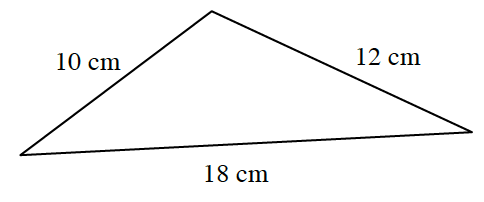### Home > PC3 > Chapter 8 > Lesson 8.1.3 > Problem8-51

8-51.Calculate the area of the triangle at right.

Use the Law of Cosines to determine the measure of one of the angles.

Use the formula below to calculate the area.

$A=\frac{1}{2}ab\sin(\theta)$